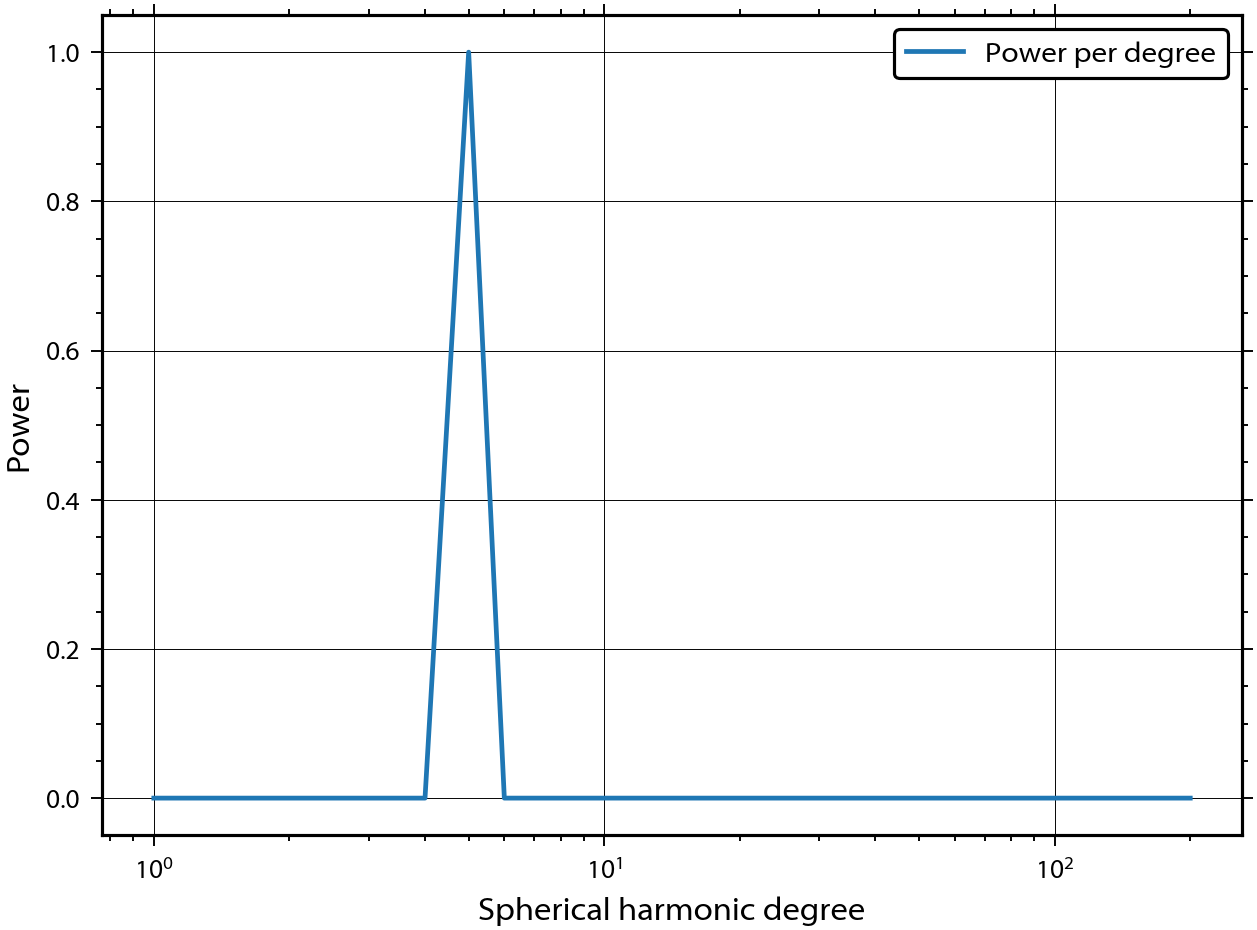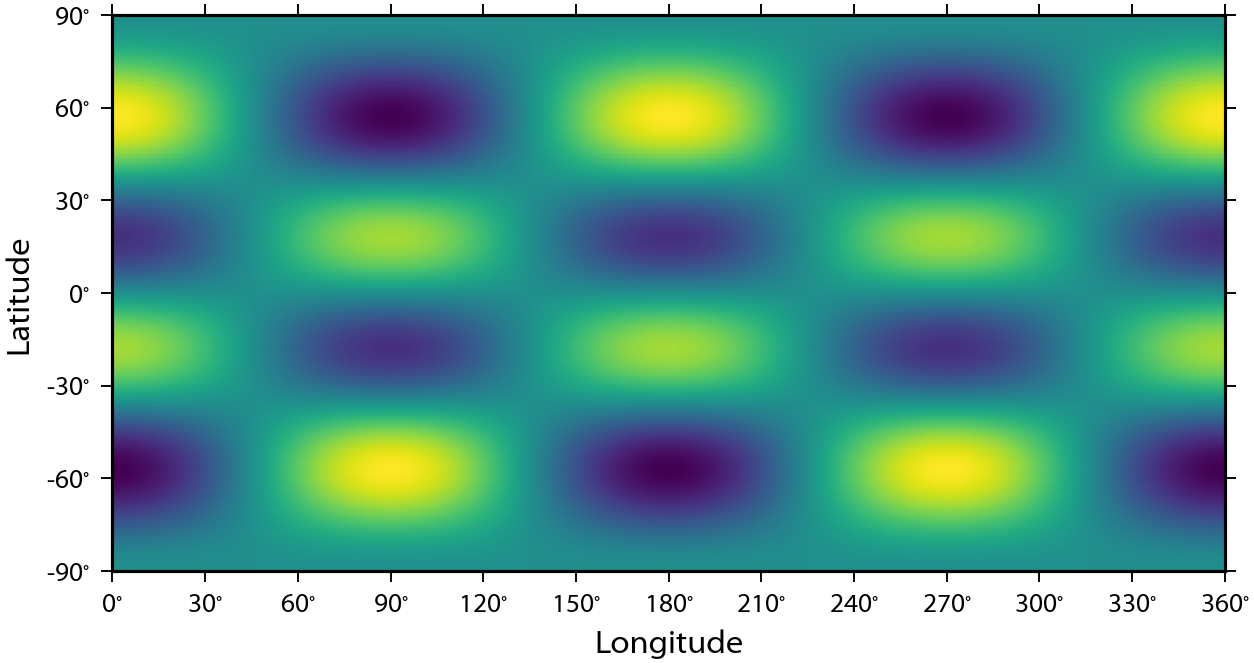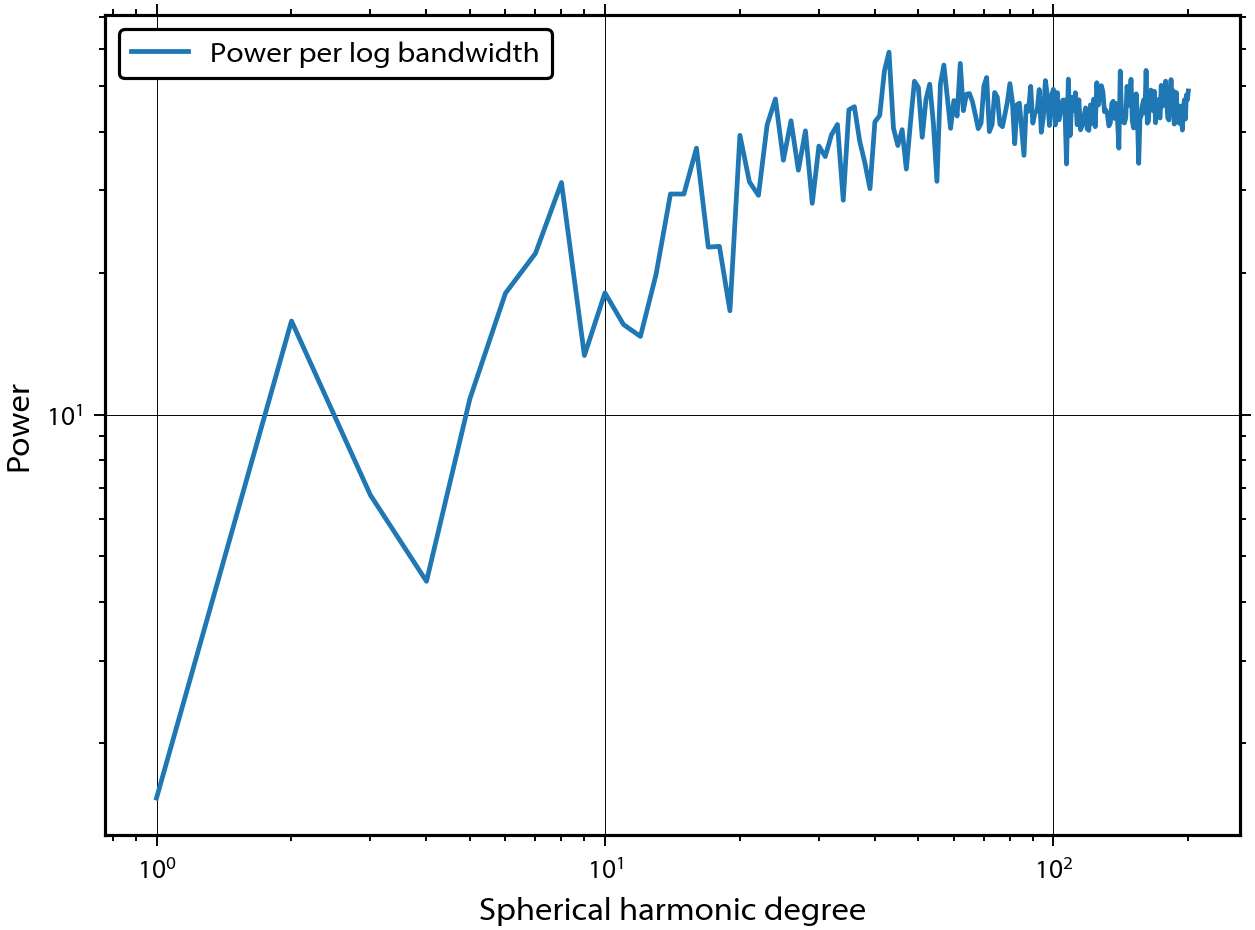# The pyshtools class interface¶

pyshtools defines three major classes that simplify access to the underlying SHTOOLS functions:

• SHCoeffs: Spherical harmonic coefficients
• SHGrid: Gridded data on the sphere
• SHWindows: Multitaper windowing functions

The underlying data are stored in internal subclasses in different formats (such as kind = 'real' or 'complex' and grid = 'DH' or 'GLQ'). In this tutorial, we will demonstrate several aspects related to the SHCoeffs and SHGrid classes.

In :
from __future__ import print_function # only necessary if using Python 2.x
%matplotlib inline

import matplotlib.pyplot as plt
import numpy as np
import pyshtools

In :
pyshtools.utils.figstyle(rel_width=0.75)
%config InlineBackend.figure_format = 'retina'  # if you are not using a retina display, comment this line


## Plot a single spherical harmonic function¶

The spherical harmonic coefficient class SHCoeffs can be initialized directly from a numpy array that holds the spherical harmonic coefficient data. Spherical harmonic coefficients are stored as a numpy array of dimension (2, lmax+1, lmax+1), where the first column corresponds to the cosine  and sine  components, the second column corresponds to the spherical harmonic degree l, and the third column corresponds to the angular order m. We will first create a numpy array of coefficients that are equal to zero, with the exception of the l=5, m=2 harmonic.

In :
lmax = 200
coeffs = np.zeros((2, lmax+1, lmax+1))
coeffs[0, 5, 2] = 1.


To initialize a new class instance, we make use of the constructor method from_array():

In :
coeffs_l5m2 = pyshtools.SHCoeffs.from_array(coeffs)


When initializing a new class instance, the default is to assume that the input coefficients are 4$\pi$ normalized excluding the Condon-Shortley phase. This normalization convention can be overridden by setting the optional parameter normalization, which takes values of '4pi', 'ortho', 'schmidt', or 'unnorm', along with the parameter csphase, which can be 1 (to exclude the Condon-Shortley phase) or -1 (to include it). The SHCoeffs class contains many methods, and here we use plot_spectrum() to plot the power spectrum:

In :
fig, ax = coeffs_l5m2.plot_spectrum(xscale='log', yscale='lin', show=False)    # show=False is used to avoid a warning when plotting in inline modeTo plot the function that corresponds to the coefficients, we first need to expand it on a grid, which can be accomplished using the expand() method:

In :
grid_l5m2 = coeffs_l5m2.expand(grid='DH2')


This returns a new SHGrid class instance. The resolution of the grid is determined automatically to correspond to the maximum degree of the spherical harmonic coefficients in order to ensure good sampling. The optional parameter grid can be 'DH2' for a Driscoll and Healy sampled grid with nlon = 2 * nlat, 'DH' for a Driscol and Healy sampled grid with nlon = nlat, or 'GLQ' for a grid used with the Gauss-Legendre quadrature expansion routines. Once the grid is created, it can be plotted using the built-in method plot().

In :
fig, ax = grid_l5m2.plot(show=False)## Initialize with a random model¶

Another constructor for the SHCoeffs class is the from_random() method. It takes a power spectrum (power per degree l of the coefficients) and generates coefficients that are independent normal distributed random variables with the provided expected power spectrum. This corresponds to a stationary and isotropic random model on the surface of the sphere whose autocorrelation function is given by the spherical harmonic addition theorem.

We initialize coefficients here with a scale-free power spectrum that has equal band power beyond the scale length that defines the size of the largest model features. The particular property of this model is that it is invariant under zoom operations.

In :
a = 20  # scale length
degrees = np.arange(lmax+1, dtype=np.float)
power = 1. / (1. + (degrees / a) ** 2) ** 0.5

coeffs_global = pyshtools.SHCoeffs.from_random(power)

fig, ax = coeffs_global.plot_spectrum(unit='per_dlogl', xscale='log', show=False)
fig2, ax2 = coeffs_global.expand(grid='DH2').plot(show=False)